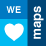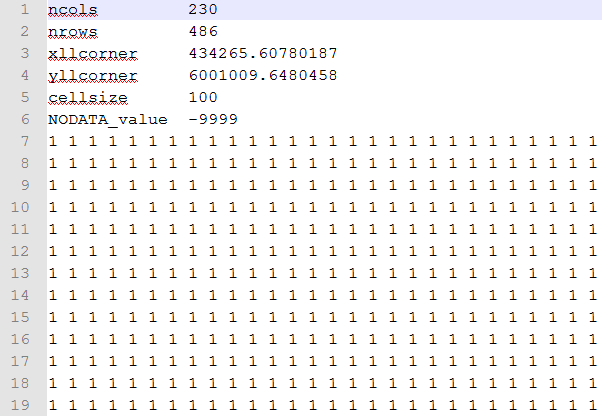# ESRI GRID file (ASC)

5190
5
04-04-2016 02:40 AMNew Contributor II

How to create an empty ESRI GRID file (ASC) based on the size of "Based polygon" with cell size 10?

1 Solution

Accepted SolutionsbyMVP Honored Contributor

I'm a little confused exactly what you're after here. ESRI GRID (raster) and ASCII (text) are two separate file formats.

You could create an ASCII file as easily as opening a text editor and start typing a file like the following, substituting your own values in the header and use the 'NODATA' value for the pixel values:5 RepliesbyMVP Esteemed Contributor

https://desktop.arcgis.com/en/arcmap/latest/tools/conversion-toolbox/an-overview-of-the-to-raster-to...

is the conversion to raster toolset

the perhaps raster to ASCII https://desktop.arcgis.com/en/arcmap/latest/tools/conversion-toolbox/raster-to-ascii.htm

By an 'empty' one... do you mean one where the bounds are classed as no data, it is not clear what you are trying to accomplishNew Contributor II

Thanks for comment, empty I mean is new Grid and it can be with no data.byMVP Honored Contributor

I'm a little confused exactly what you're after here. ESRI GRID (raster) and ASCII (text) are two separate file formats.

You could create an ASCII file as easily as opening a text editor and start typing a file like the following, substituting your own values in the header and use the 'NODATA' value for the pixel values:byMVP Esteemed Contributor

well you could exploit Darren's data and simply convert the nodata value to 1, then use the

ASCII to Raster—Help | ArcGIS for Desktop .  You will obviously have to have the correct number of rows and columns and properly specify the location of the bottom left cell (center or corner) and the X and Y cell size (ie 10m for both)

You can cheat even further by using the ideas here.

NumPyArrayToRaster—Help | ArcGIS for Desktop

```import numpy as np
import arcpy
LLcorner = arcpy.Point(500000,5000000) # x,y coordinate of the lower left corner, in this case the middle of a UTM zone
rows= 10
cols = 10
cell_size = 10
nodata = 0.0 # or whatever
a = np.zeros((rows,cols),dtype='float64') # or 'int32'
out_raster = arcpy.NumPyArrayToRaster( a, LLcorner, x_cell_size = cell_size, value_to_nodata = nodata)
out_raster.save(" path and name to raster) # specify and extension for non-esri grid formats such as tif```New Contributor II

Thanks all for that. Now the step 2 I wish to achieve is below:

Step 2

Once the ASC file in #1 above is created, I need to run the following formula on each of the grid cell.

First:

With reference from the point of interest, as shown in the "Based polygon" image below (with green square),

I want to run   tan-1 (x/y)

(Grid point (x,y) will be in the center of each cell)

For Example:

Location of point of interest (0, 0)

tan-1 of (75, 50) = 56.3 (value of one of the grid cell with reference from point of interest (0,0))

[If point of interest is 10, 10 in the map, then the X,Y calculated above will be 85, 60 in the map]

Second:

I need to calculate linear interpolation (which is not yet confirmed the formula)

Third:

Result A x Result B x [Some other value]I am trying to figure out if there is other way to do, but thanks all in advance for reply.